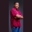Related Tags

c#
communitycreator

# How to calculate the perimeter of a circle in C#Theodore Kelechukwu Onyejiaku

## Overview

The perimeter of a circle is the total distance around it. Another word for it is the circumference. The product of Pi (π), its radius, and 2 give us the perimeter of a circle.

We can easily calculate the perimeter of a circle in C#.

### Syntax

2 * Math.PI * r
Syntax for finding the perimeter of a circle in C#

### Variables

• Math.PI: This is an inbuilt constant value for the mathematical Pi in C#.
• r: This is the radius of the circle.

### Return value

The value returned is a double value, representing the total distance around the circle.

### Example

using System;
class CirclePerimeter
{
static void Main()
{

// Cet the perimeters of the circles
double per1 = 2 * Math.PI * rad1;
double per2 = 2 * Math.PI * rad2;
double per3 = 2 * Math.PI * rad3;
double per4 = 2 * Math.PI * rad4;

// Print the perimeters
}
}
Getting the perimeter of a circle in C#

### Explanation

• Lines 7–10: We create some radius values.
• Lines 13–16: We get the perimeters of the circles with the created radius values.
• Lines 19–22: We print the perimeters.

RELATED TAGS

c#
communitycreator

CONTRIBUTORTheodore Kelechukwu Onyejiaku
RELATED COURSES

View all Courses

Keep Exploring

Learn in-demand tech skills in half the time